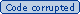# Implementation

Training:

• Compute Gram matrix Gij = yiyjK(qi; qj)
• Solve QP to get a
• Compute intercept c by using complementarity or duality

Classification:

• Compute ki = K(q, qi) for support vectors qi
• Compute f = ci aikiyi
• Test sign(f)